# Working with Microsoft Equation

Word
An equation is a mathematical statement that two mathematical expressions are equal (indicated by the sign =). Microsoft Equation helps you type fractions, exponents, integrals, and so on in Word documents. In addition, Word allows you to insert an equation from the Gallery, create a new equation, edit, change, or delete a part or the entire equation.

## Insert an equation in the Word document

To insert an equation to the Word document, on the Insert tab, in the Symbols group, click the arrow next to the Equation button: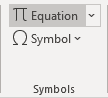You can use the vertical scroll bar in the Gallery to display additional equations (how to add an equation into the Gallery, see How to add your own equation to the Equation gallery). If you see what you want, click it to insert it at the current insertion point in the document. If you add it into an empty paragraph, the equation is rendered in Display mode. If the equation is on the same line as text, it is rendered in Inline mode: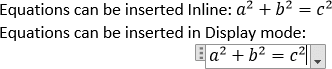## Create an equation

To add a new equation, do one of the following:

• On the Insert tab, in the Symbols group, click the arrow next to Equation, and then click Insert New Equation,
• On the Insert tab, in the Symbols group, click the Equation button,
• Or simply press Alt+=: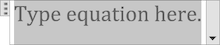## Working with equations in Word

Word for Microsoft 365 opens the Equation tab: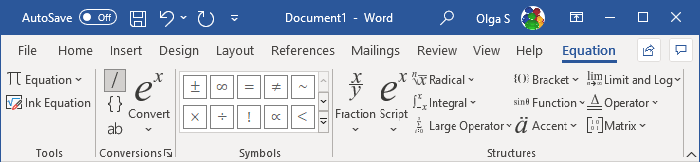Word for Microsoft 365 provides two formats of equations: Professional and Linear: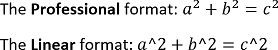By default, Word uses the Professional representation, but if you need Linear, do one of the following:

• Select the equation(s) you want to change, on the Equation tab, in the Conversions group, in the Convert drop-down list, choose the appropriate option: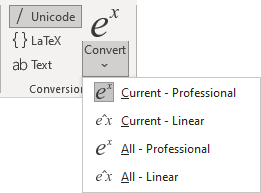• Click the down arrow to the left of the equation, then select the appropriate option from the context menu: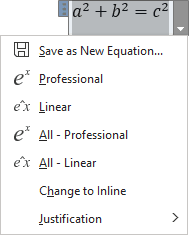• The Equation tab contains dozens of equation templates. Within each button on the toolbar, there are several tools available. Simply click on a button to see the tools contained in each.

## Example of equations – step-by-step guides

How to create an equation step-by-step, see:

For more details about equations, see Setting font size and styles in an equation and Adjusting spacing and alignment in an equation.

See also this tip in French: Comment insérer des équations dans Microsoft Word.